## Breaking

CustomWritings.com - get an essay written for you by academic experts. Feel free to get writing assistance online.

## RD Sharma Solutions for Class 8 Chapter 16 Understanding Shapes- II (Quadrilaterals) Free Online

EXERCISE 16.1 PAGE NO: 16.15
1. Define the following terms:
Solution:
Definition: Let A, B, C and D be four points in a plane such that: (a) no three of them are collinear. (b) The line segments AB, BC, CD and DA do not intersect except at their end points. Then an Enclosed figure with four sides is termed as Quadrilateral.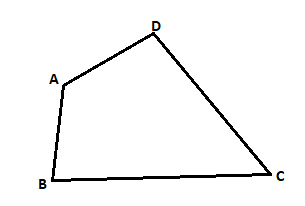Definition: If the line containing any side of the quadrilateral has the remaining vertices on the same side of it is termed as Convex Quadrilateral.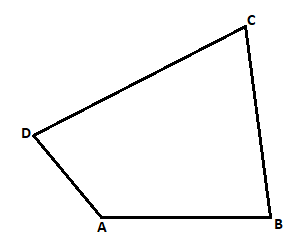Vertices A, B lie on the same side of line CD, vertices B, C lie on the same side of line DA, vertices C, D lie on the same side of line AB, vertices D, A lie on the same side of line BC.
2. In a quadrilateral, define each of the following:
(i) Sides
(ii) Vertices
(iii) Angles
(iv) Diagonals
(vii) Opposite sides
(viii) Opposite angles
(ix) Interior
(x) Exterior
Solution: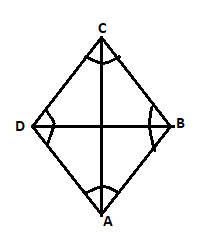(i) Sides: In a quadrilateral. All the sides may have same of different length.
The four line segments AB, BC, CD and DA are called its sides.
(ii) Vertices
Vertices are the angular points where two sides or edges meet.
A, B, C and D are the four vertices in a quadrilateral.
(iii) Angles
Angle is the inclination between two sides of a quadrilateral. i.e. meeting point of two sides is an angle. ABC, BCA, CDA and DAB are the four angles in a quadrilateral.
(iv) Diagonals
The lines joining two opposite vertices is called the diagonals in a quadrilateral.
BD and AC are the two diagonals.
Angles having one common arm onto the sides is called the adjacent angles.
ABC, BCD are adjacent angles in a quadrilateral.
When two sides have common endpoint is termed as adjacent sides.
AB BC, BC CA, CD DA, DA AB are pairs of adjacent sides in a quadrilateral.
(vii) Opposite sides: Opposite sides when they don’t meet at any point is termed as opposite sides.
AB CD, BC DA are the pairs of opposite sides in a quadrilateral.
(viii) Opposite angles
Two angles, which are not adjacent angles are termed as opposite angles.
A and C, angles B and D are opposite angles in a quadrilateral.
(ix) Interior
The part of plane when points are enclosed within the quadrilateral is called as interior.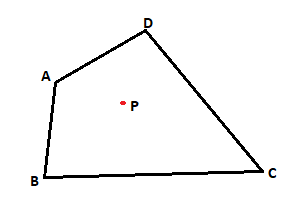(x) Exterior
The part of plane when points are not enclosed within the quadrilateral is called as exterior.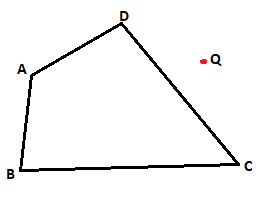3. Complete each of the following, so as to make a true statement:
(i) A quadrilateral has ________ sides.
(ii) A quadrilateral has ________angles.
(iii) A quadrilateral has ________, no three of which are ________.
(iv) A quadrilateral has ________diagonals.
(v) The number of pairs of adjacent angles of a quadrilateral is ________.
(vi) The number of pairs of opposite angles of a quadrilateral is ________.
(vii) The sum of the angles of a quadrilateral is ________.
(viii) A diagonal of a quadrilateral is a line segment that joins two ________ vertices of the quadrilateral.
(ix) The sum of the angles of a quadrilateral is ________ right angles.
(x) The measure of each angle of a convex quadrilateral is ________ 180°.
(xi) In a quadrilateral the point of intersection of the diagonals lies in ________ of the quadrilateral.
(xii) A point is in the interior of a convex quadrilateral, if it is in the ________ of its two opposite angles.
(xiii) A quadrilateral is convex if for each side, the remaining ________ lie on the same side of the line containing the side.
Solution:
(i) A quadrilateral has four sides.
(ii) A quadrilateral has four angles.
(iii) A quadrilateral has four, no three of which are collinear.
(iv) A quadrilateral has two diagonals.
(v) The number of pairs of adjacent angles of a quadrilateral is four.
(vi) The number of pairs of opposite angles of a quadrilateral is two.
(vii) The sum of the angles of a quadrilateral is 3600.
(viii) A diagonal of a quadrilateral is a line segment that joins two opposite vertices of the quadrilateral.
(ix) The sum of the angles of a quadrilateral is four right angles.
(x) The measure of each angle of a convex quadrilateral is less than 180°.
(xi) In a quadrilateral the point of intersection of the diagonals lies in interior of the quadrilateral.
(xii) A point is in the interior of a convex quadrilateral, if it is in the interiors of its two opposite angles.
(xiii) A quadrilateral is convex if for each side, the remaining vertices lie on the same side of the line containing the side.
4. In Fig. 16.19, ABCD is a quadrilateral.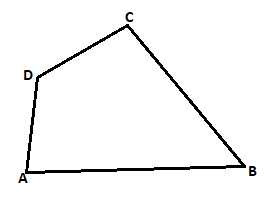(i) Name a pair of adjacent sides.
(ii) Name a pair of opposite sides.
(iii) How many pairs of adjacent sides are there?
(iv) How many pairs of opposite sides are there?
(v) Name a pair of adjacent angles.
(vi) Name a pair of opposite angles.
(vii) How many pairs of adjacent angles are there?
(viii) How many pairs of opposite angles are there?
Solution:
(i) Name a pair of adjacent sides.
Adjacent sides are: AB, BC or BC, CD or CD, DA or AD, AB
(ii) Name a pair of opposite sides.
opposite sides are: AB, CD or BC, DA
(iii) How many pairs of adjacent sides are there?
Four pairs of adjacent sides i.e. AB BC, BC CD, CD DA and DA AB
(iv) How many pairs of opposite sides are there?
Two pairs of opposite sides. AB, DC and DA, BC
(v) Name a pair of adjacent angles.
Four pairs of Adjacent angles are: D∠AB A∠BC, A∠BC B∠CA, B∠CA C∠DA or C∠DA D∠AB
(vi) Name a pair of opposite angles.
Four pair of opposite angles are: D∠AB B∠CA and A∠BC C∠DA
(vii) How many pairs of adjacent angles are there?
Four pairs of adjacent angles. D∠AB A∠BC, A∠BC B∠CA, B∠CA C∠DA and C∠DA D∠AB
(viii) How many pairs of opposite angles are there?
Two pairs of opposite angles. D∠AB B∠CA and A∠BC C∠DA
5. The angles of a quadrilateral are 110°, 72°, 55° and x°. Find the value of x.
Solution:
We know that Sum of angles of a quadrilateral is = 360°
So,
110° + 72° + 55° + x° = 360°
x° = 360° – 237°
x° = 123o
∴ Value of x is 123o
6. The three angles of a quadrilateral are respectively equal to 110°, 50° and 40°. Find its fourth angle.
Solution:
We know that Sum of angles of a quadrilateral is = 360°
So,
110° + 50° + 40° + x° = 360°
x° = 360° – 200°
x° = 160o
∴ Value of fourth angle is 160o
7. A quadrilateral has three acute angles each measures 80°. What is the measure of the fourth angle?
Solution:
We know that Sum of angles of a quadrilateral is = 360°
So,
80° + 80° + 80° + x° = 360°
x° = 360° – 240°
x° = 120o
∴ Value of fourth angle is 120o
8. A quadrilateral has all its four angles of the same measure. What is the measure of each?
Solution:
We know that Sum of angles of a quadrilateral is = 360°
Let each angle be xo
So,
xo + xo + xo + xo = 360o
xo = 360o/4
= 90o
∴ Value of angle is 90o each.
9. Two angles of a quadrilateral are of measure 65° and the other two angles are equal. What is the measure of each of these two angles?
Solution:
We know that Sum of angles of a quadrilateral is = 360°
Let each angle be xo
So,
65o + 65o + xo + xo = 360o
2xo = 360o – 130o
x= 230o/2
= 115o
∴ Value of two angles is 115o each.
10. Three angles of a quadrilateral are equal. Fourth angle is of measure 150°. What is the measure of equal angles?
Solution:
We know that Sum of angles of a quadrilateral is = 360°
Let each angle be xo
So,
150o + xo + xo + xo = 360o
3xo = 360o – 150o
x= 210o/3
= 70o
∴ Value of equal angles is 70o each.
11. The four angles of a quadrilateral are as 3 : 5 : 7 : 9. Find the angles.
Solution:
We know that Sum of angles of a quadrilateral is = 360°
Let each angle be xo
So,
3xo + 5xo + 7xo + 9xo = 360o
24xo = 360o
x= 360o/24
= 15o
Value of angles are
3x = 3 × 15 = 45o
5x = 5 × 15 = 75o
7x = 7 × 15 = 105o
9x = 9 × 15 = 135o
∴ Value of angles are 45o, 75o, 105o, 135o
12. If the sum of the two angles of a quadrilateral is 180°. What is the sum of the remaining two angles?
Solution:
We know that Sum of angles of a quadrilateral is = 360°
Let the sum of two angles be 180o
Let angle be xo
So,
180o + xo = 360o
xo = 360o – 180o
x= 180o
∴ Sum of remaining two angles is 180o
13. In Figure, find the measure of ∠MPN.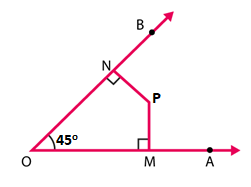Solution:
We know that Sum of angles of a quadrilateral is = 360°
In the quadrilateral MPNO
∠NOP = 45°, ∠OMP = ∠PNO = 90°
Let angle ∠MPN is x°
∠NOP + ∠OMP + ∠PNO + ∠MPN = 360°
45° + 90° + 90° + x° = 360°
x° = 360° – 225°
x° = 135°
∴ Measure of ∠MPN is 135°
14. The sides of a quadrilateral are produced in order. What is the sum of the four exterior angles?
Solution: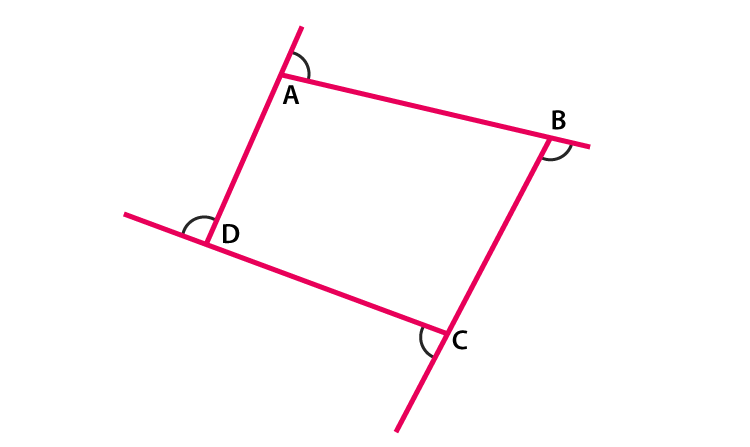We know that, exterior angle + interior adjacent angle = 180° [Linear pair]
Applying relation for polygon having n sides
Sum of all exterior angles + Sum of all interior angles = n × 180°
Sum of all exterior angles = n × 180° – Sum of all interior angles
= n × 180° – (n -2) × 180° [Sum of interior angles is = (n – 2) x 180°]
= n × 180° – n × 180° + 2 × 180°
= 180°n – 180°n + 360°
= 360°
∴ Sum of four exterior angles is 360o
15. In Figure, the bisectors of ∠A and ∠B meet at a point P. If ∠C =100° and ∠D = 50°, find the measure of ∠APB.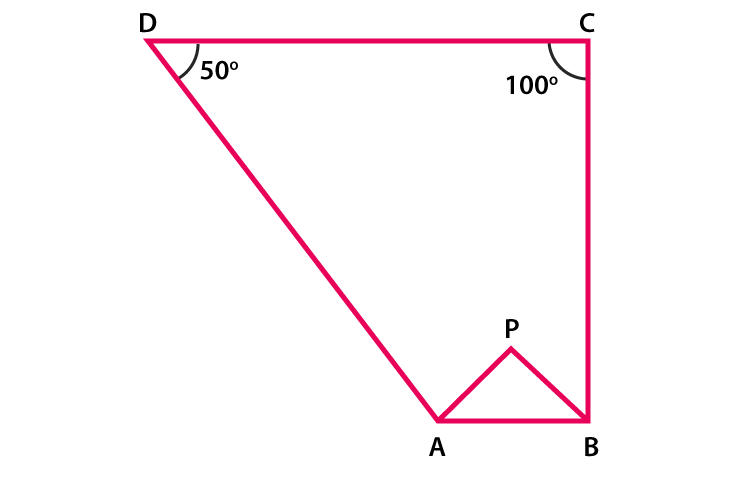Solution:
We know that Sum of angles of a quadrilateral is = 360°
In the quadrilateral ABCD
Given, ∠C =100° and ∠D = 50°
∠A + ∠B + ∠C + ∠D = 360o
∠A + ∠B + 100o + 50o = 360o
∠A + ∠B = 360o – 150o
∠A + ∠B = 210o ……. (Equation 1)
Now in Δ APB
½ ∠A + ½ ∠B + ∠APB = 180o (since, sum of triangle is 180o)
∠APB = 180o – ½ (∠A + ∠B)………. (Equation 2)
On substituting value of ∠A + ∠B = 210 from equation (1) in equation (2)
∠APB = 180o – ½ (210o)
= 180o – 105o
= 75o
∴ The measure of ∠APB is 75o
16. In a quadrilateral ABCD, the angles A, B, C and D are in the ratio 1 : 2 : 4 : 5. Find the measure of each angle of the quadrilateral.
Solution:
We know that Sum of angles of a quadrilateral is = 360°
Let each angle be xo
So,
xo + 2xo + 4xo + 5xo = 360o
12xo = 360o
x= 360o/12
= 30o
Value of angles are
x = 30o
2x = 2 × 30 = 60o
4x = 4 × 30 = 120o
5x = 5 × 30 = 150o
∴ Value of angles are 30o, 60o, 120o, 150o
17. In a quadrilateral ABCD, CO and DO are the bisectors of ∠C and ∠D respectively. Prove that ∠COD = 1/2 (∠A +∠B).
Solution:
We know that sum of angles of a quadrilateral is 360°
In the quadrilateral ABCD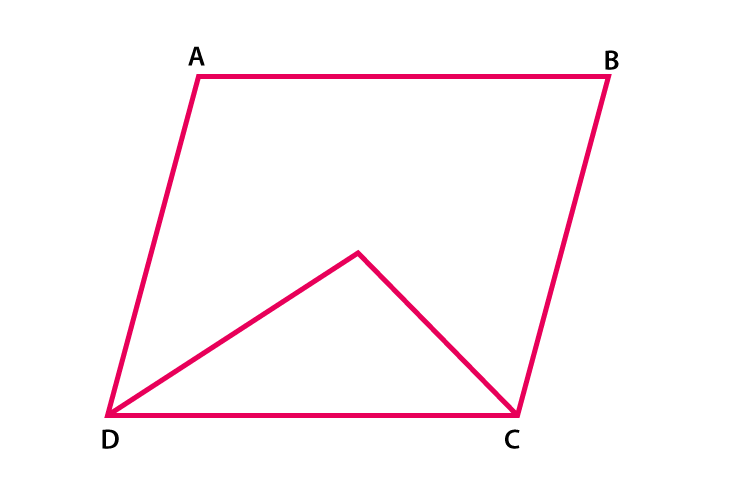∠A + ∠B + ∠C + ∠D = 360o
∠A + ∠B = 360o – (∠C + ∠D)
½ (∠A + ∠B) = ½ [360o – (∠C + ∠D)]
= 180o – ½ (∠C + ∠D)]……… (Equation 1)
Now in Δ DOC
½ ∠D + ½ ∠C + ∠COD = 180o (since sum of triangle = 180o)
½ (∠C + ∠D) + ∠COD = 180o
∠COD = 180o – ½ (∠C + ∠D)………. (Equation 2)
From above equations (1) and (2) RHS is equal, then LHS will also be equal.
∴ ∠COD = ½ (∠A + ∠B) is proved.
18. Find the number of sides of a regular polygon, when each of its angles has a measure of
(i) 160°
(ii) 135°
(iii) 175°
(iv) 162°
(v) 150°
Solution:
The measure of interior angle A of a polygon of n sides is given by A = [(n-2) ×180o]/n
(i) 160o
Angle of quadrilateral is 160°
160o = [(n-2) ×180o]/n
160on = (n-2) ×180o
160on = 180on – 360o
180on – 160o = 360o
20on = 360o
n = 360o/20
= 18
∴ Number of sides are 18
(ii) 135o
Angle of quadrilateral is 135°
135o = [(n-2) ×180o]/n
135on = (n-2) ×180o
135on = 180on – 360o
180on – 135o = 360o
45on = 360o
n = 360o/45
= 8
∴ Number of sides are 8
(iii) 175o
Angle of quadrilateral is 175°
175o = [(n-2) ×180o]/n
175on = (n-2) ×180o
175on = 180on – 360o
180on – 175o = 360o
5on = 360o
n = 360o/5
= 72
∴ Number of sides are 72
(iv) 162o
Angle of quadrilateral is 162°
162o = [(n-2) ×180o]/n
162on = (n-2) ×180o
162on = 180on – 360o
180on – 162o = 360o
18on = 360o
n = 360o/18
= 20
∴ Number of sides are 20
(v) 150o
Angle of quadrilateral is 160°
150o = [(n-2) ×180o]/n
150on = (n-2) ×180o
150on = 180on – 360o
180on – 150o = 360o
30on = 360o
n = 360o/30
= 12
∴ Number of sides are 12
19. Find the numbers of degrees in each exterior angle of a regular pentagon.
Solution:
We know that the sum of exterior angles of a polygon is 360°
Measure of each exterior angle of a polygon is = 360o/n , where n is the number of sides
We know that number of sides in a pentagon is 5
Measure of each exterior angle of a pentagon is = 360o/5 = 72o
∴ Measure of each exterior angle of a pentagon is 72o
20. The measure of angles of a hexagon are x°, (x-5)°, (x-5)°, (2x-5)°, (2x-5)°, (2x+20)°. Find value of x.
Solution:
By using the formula,
The sum of interior angles of a polygon = (n – 2) × 180°, (where n = number of sides of polygon.)
We know, a hexagon has 6 sides. So,
The sum of interior angles of a hexagon = (6 – 2) × 180° = 4 × 180° = 720°
x°+ (x-5)°+ (x-5)°+ (2x-5)°+ (2x-5)°+ (2x+20)° = 720°
x°+ x°- 5°+ x° – 5°+ 2x° – 5°+ 2x° – 5°+ 2x° + 20° = 720°
9x° = 720°
x = 720o/9
= 80o
∴ Value of x is 80o
21. In a convex hexagon, prove that the sum of all interior angle is equal to twice the sum of its exterior angles formed by producing the sides in the same order.
Solution:
By using the formulas,
The sum of interior angles of a polygon = (n – 2) × 180°
The sum of interior angles of a hexagon = (6 – 2) × 180° = 4 × 180° = 720°
The Sum of exterior angle of a polygon is 360°
∴ Sum of interior angles of a hexagon = twice the sum of interior angles.
Hence proved.
22. The sum of the interior angles of a polygon is three times the sum of its exterior angles. Determine the number of sides of the polygon.
Solution:
By using the formulas,
The sum of interior angles of a polygon = (n – 2) × 180° …..(i)
The Sum of exterior angle of a polygon is 360°
So,
Sum of interior angles = 3 × sum of exterior angles
= 3 × 360° = 1080°…..(ii)
Now by equating (i) and (ii) we get,
(n – 2) × 180° = 1080°
n – 2 = 1080o/180o
n – 2 = 6
n = 6 + 2
= 8
∴ Number of sides of a polygon is 8
23. Determine the number of sides of a polygon whose exterior and interior angles are in the ratio 1 : 5.
Solution:
By using the formulas,
The sum of interior angles of a polygon = (n – 2) × 180° ……..(i)
The Sum of exterior angle of a polygon is 360°
We know that Sum of exterior angles/Sum of interior angles = 1/5…..(ii)
So, equating (i) and (ii) we get
360o/(n – 2) × 180° = 1/5
On cross multiplication,
(n – 2) × 180° = 360o × 5
(n – 2) × 180° = 1800o
(n – 2) = 1800o/180o
(n – 2) = 10
n = 10 + 2
= 12
∴ Numbers of sides of a polygon is 12
24. PQRSTU is a regular hexagon, determine each angle of ΔPQT.
Solution:
We know that the sum of interior angles of a polygon = (n – 2) × 180°
The sum of interior angles of a hexagon = (6 – 2) × 180° = 4 × 180° = 720°
Measure of each angle of hexagon = 720o/6 = 120o
∠PUT = 120o Proved.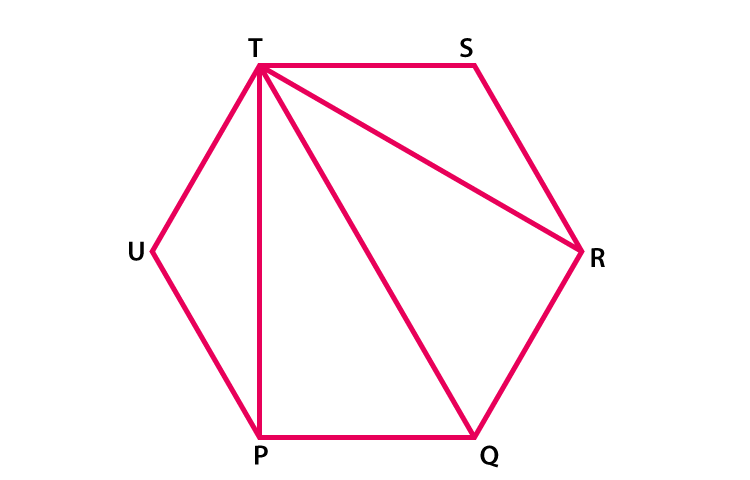In Δ PUT
∠PUT + ∠UTP + ∠TPU = 180o (sum of triangles)
120o + 2∠UTP = 180o (since Δ PUT is an isosceles triangle )
2∠UTP = 180o – 120o
2∠UTP = 60o
∠UTP = 60o/2
= 30o
∠UTP = ∠TPU = 30o similarly ∠RTS = 30o
∴ ∠PTR = ∠UTS – ∠UTP – ∠RTS
= 120o – 30o – 30o
= 60o
∠TPQ = ∠UPQ – ∠UPT
= 120o – 30o
= 90o
∠TQP = 180o – 150o
= 30o (by using angle sum property of triangle in ΔPQT)
∴ ∠P = 90o, ∠Q = 60o, ∠T = 30o
Courtesy : CBSE